# NCERT Solutions for Class 9 Maths Chapter 4 Exercise 4.2 – Linear Equations in two Variables

Download NCERT Solutions for Class 9 Maths Chapter 4 Exercise 4.2 – Linear Equations in two Variables. This Exercise contains 3 questions, for which detailed answers have been provided in this note. In case you are looking at studying the remaining Exercise for Class 9 for Maths NCERT solutions for Chapter 4 or other Chapters, you can click the link at the end of this Note.

NCERT Solutions for Class 9 Maths Chapter 4 Exercise 4.2 – Linear Equations in two Variables – Video Explanation

### NCERT Solutions for Class 9 Maths Chapter 4 Exercise 4.2 – Linear Equations in two Variables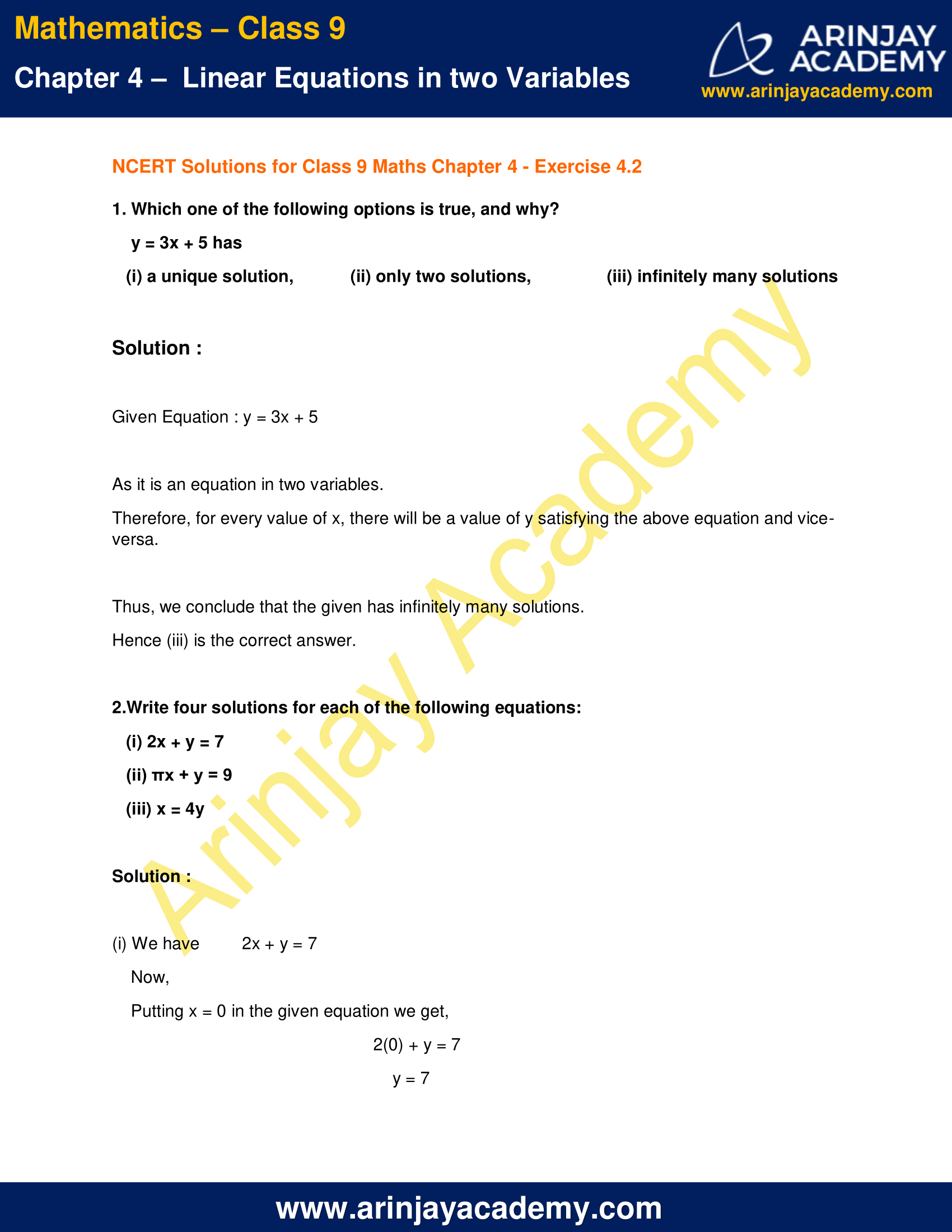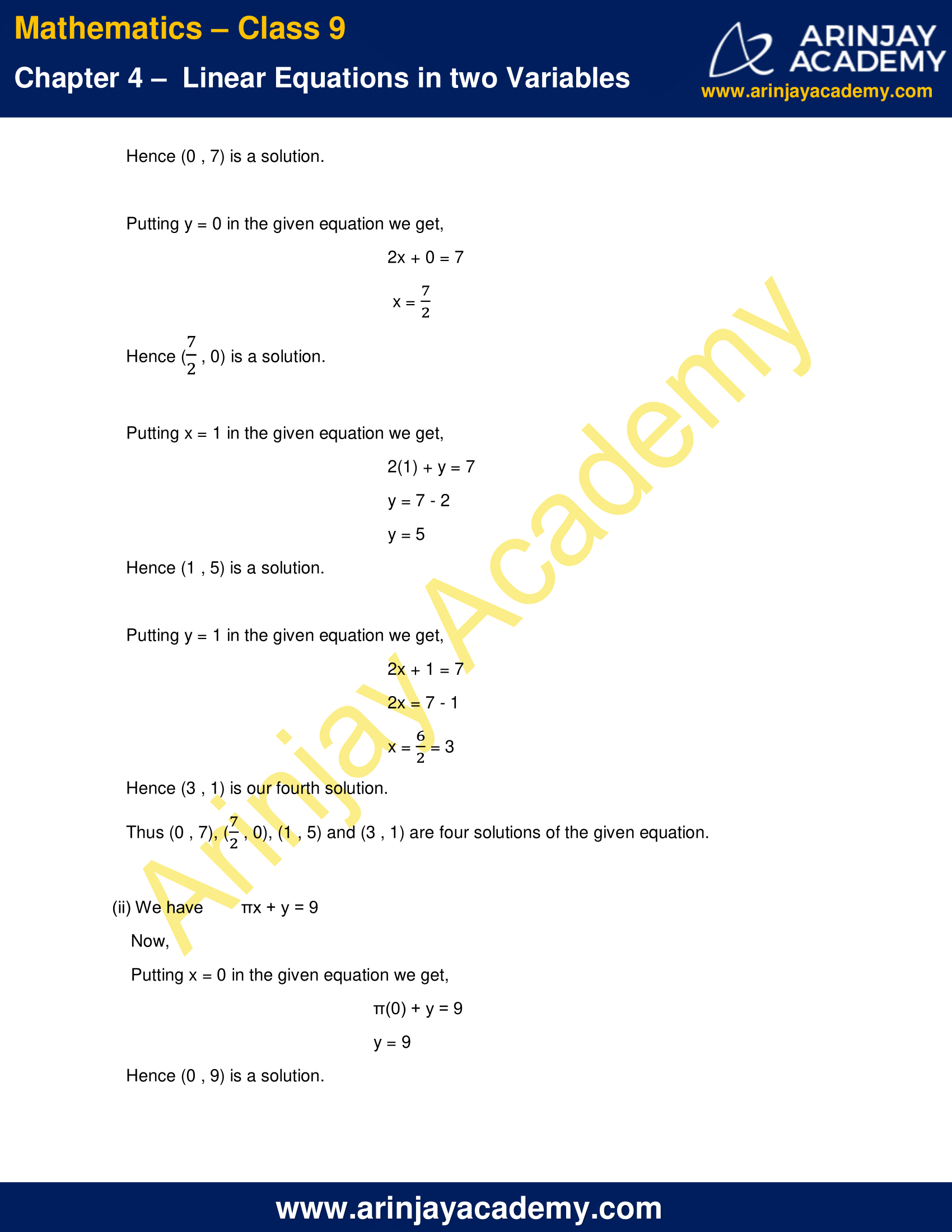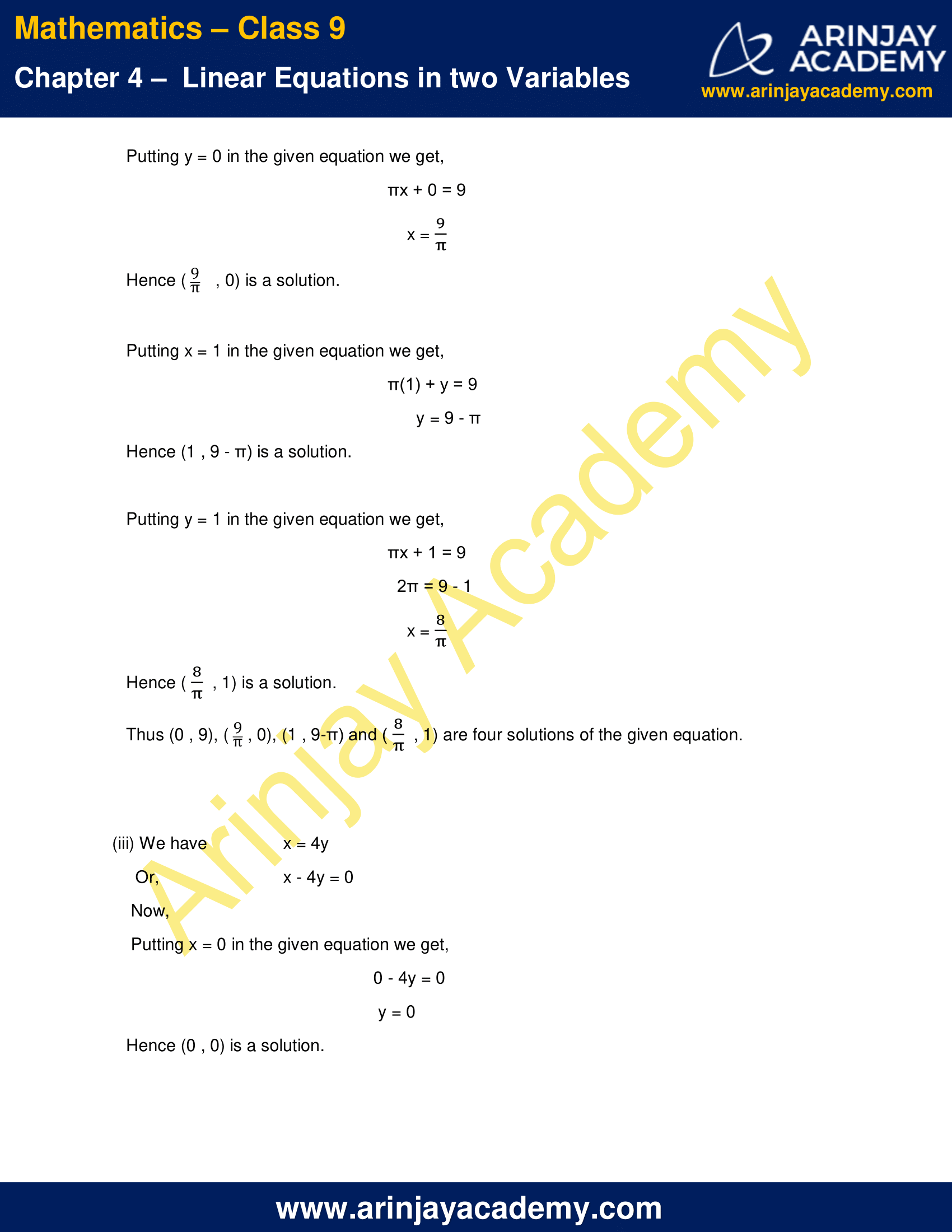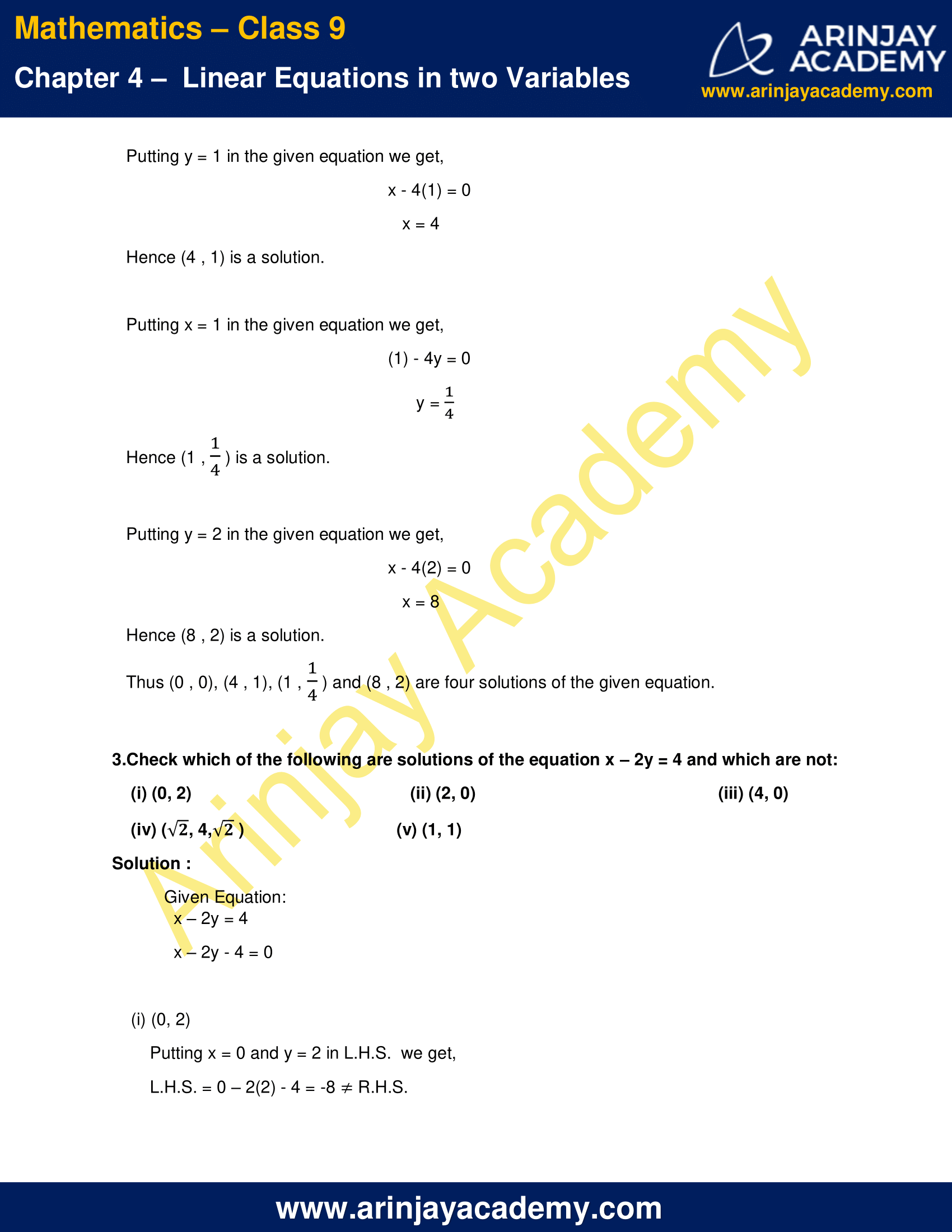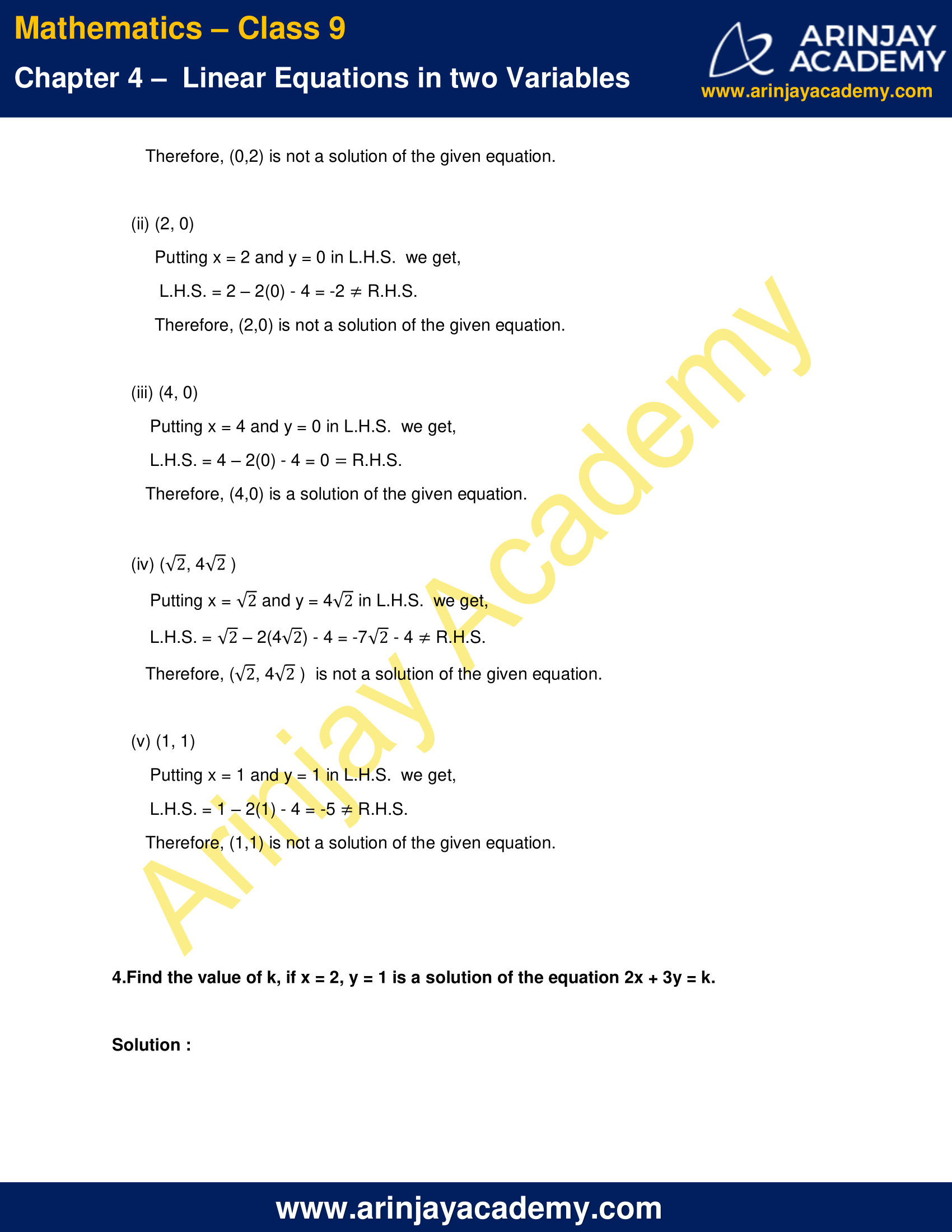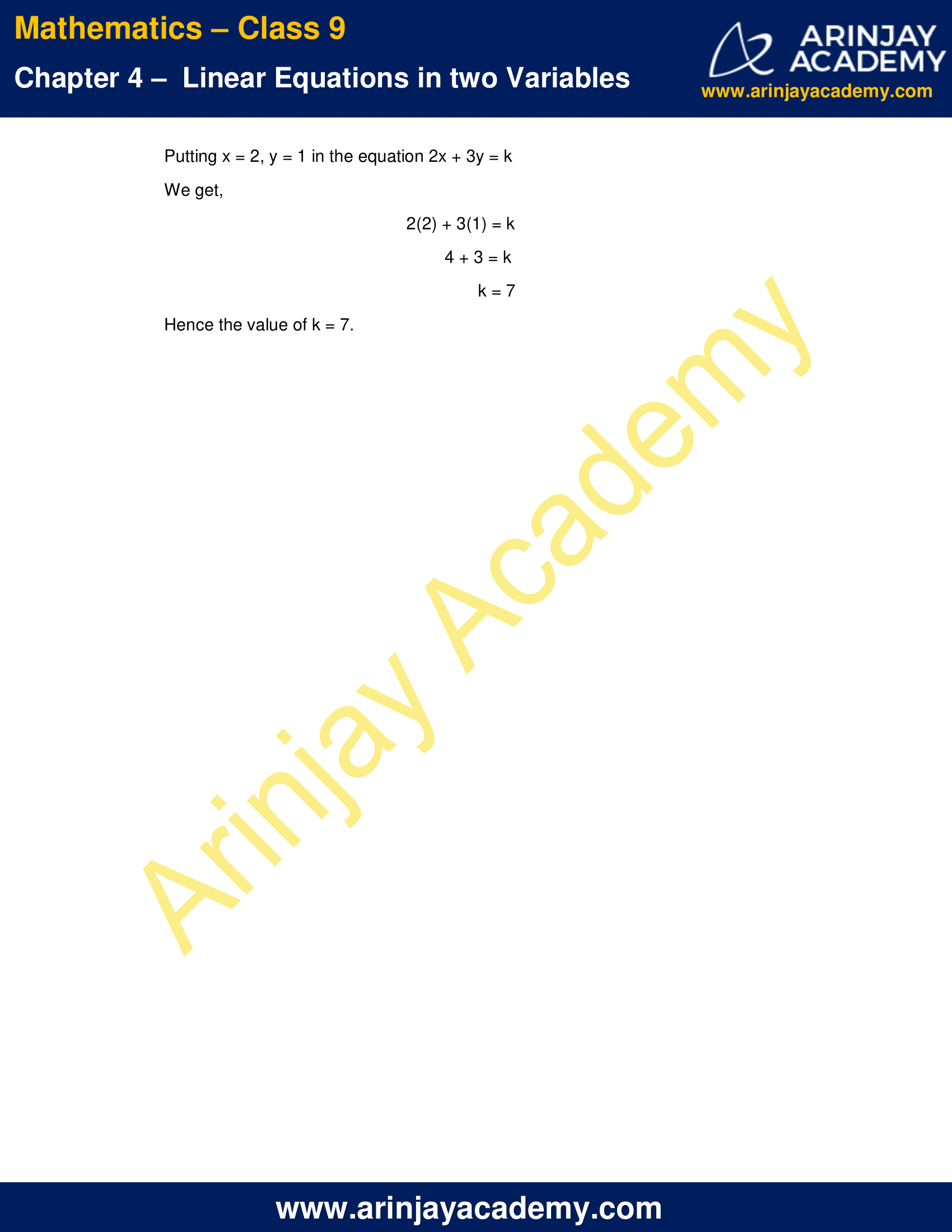NCERT Solutions for Class 9 Maths Chapter 4 Exercise 4.2 – Linear Equations in two Variables

1. Which one of the following options is true, and why?
y = 3x + 5 has
(i) a unique solution,
(ii) only two solutions,
(iii) infinitely many solutions

Solution :

Given Equation : y = 3x + 5
As it is an equation in two variables.
Therefore, for every value of x, there will be a value of y satisfying the above equation and vice-versa.
Thus, we conclude that the given has infinitely many solutions.
Hence (iii) is the correct answer.

2.Write four solutions for each of the following equations:
(i) 2x + y = 7
(ii) πx + y = 9
(iii) x = 4y

Solution :

(i) We have, 2x + y = 7

Putting x = 0 in the given equation we get,
2(0) + y = 7
y = 7
Hence (0 , 7) is a solution.

Putting y = 0 in the given equation we get,
2x + 0 = 7
x = 7/2
Hence (7/2 , 0) is a solution.

Putting x = 1 in the given equation we get,
2(1) + y = 7
y = 7 – 2
y = 5
Hence (1 , 5) is a solution.

Putting y = 1 in the given equation we get,
2x + 1 = 7
2x = 7 – 1
x = 6/2 = 3
Hence (3 , 1) is our fourth solution.

Thus (0 , 7), (7/2 , 0), (1 , 5) and (3 , 1) are four solutions of the given equation.

(ii) We have, πx + y = 9

Putting x = 0 in the given equation we get,
π(0) + y = 9
y = 9
Hence (0 , 9) is a solution.

Putting y = 0 in the given equation we get,
πx + 0 = 9
x = (9/π)
Hence (9/π, 0) is a solution.

Putting x = 1 in the given equation we get,
π(1) + y = 9
y = 9 – π
Hence (1 , 9 – π) is a solution.

Putting y = 1 in the given equation we get,
πx + 1 = 9
2π = 9 – 1
x = 8/π
Hence (8/π , 1) is a solution.

Thus (0 , 9), (9/π , 0), (1 , 9-π) and (8/π, 1) are four solutions of the given equation.

(iii) We have, x = 4y
Or,  x – 4y = 0

Putting x = 0 in the given equation we get,
0 – 4y = 0
y = 0
Hence (0 , 0) is a solution.

Putting y = 1 in the given equation we get,
x – 4(1) = 0
x = 4
Hence (4 , 1) is a solution.

Putting x = 1 in the given equation we get,
(1) – 4y = 0
y = 1/4
Hence (1 , 1/4) is a solution.

Putting y = 2 in the given equation we get,
x – 4(2) = 0
x = 8
Hence (8 , 2) is a solution.

Thus (0 , 0), (4 , 1), (1 , 1/4) and (8 , 2) are four solutions of the given equation.

3.Check which of the following are solutions of the equation x – 2y = 4 and which are not:

(i) (0, 2)
(ii) (2, 0)
(iii) (4, 0)
(iv) (√2, 4√2 )
(v) (1, 1)

Solution :

Given Equation:
x – 2y = 4
x – 2y – 4 = 0

(i) (0, 2)

Putting x = 0 and y = 2 in L.H.S.  we get,
L.H.S. = 0 – 2(2) – 4 = -8  R.H.S.
Therefore, (0,2) is not a solution of the given equation.

(ii) (2, 0)

Putting x = 2 and y = 0 in L.H.S.  we get,
L.H.S. = 2 – 2(0) – 4 = -2  R.H.S.
Therefore, (2,0) is not a solution of the given equation.

(iii) (4, 0)

Putting x = 4 and y = 0 in L.H.S.  we get,
L.H.S. = 4 – 2(0) – 4 = 0  R.H.S.
Therefore, (4,0) is a solution of the given equation.

(iv) ( √2 , 4√2 )

Putting x = √2 and y = 4√2 in L.H.S.  we get,
L.H.S. = √2 – 2(4√2) – 4 = -7√2 – 4  R.H.S.
Therefore, ( √2, 4√2 )  is not a solution of the given equation.

(v) (1, 1)

Putting x = 1 and y = 1 in L.H.S.  we get,
L.H.S. = 1 – 2(1) – 4 = -5  R.H.S.
Therefore, (1,1) is not a solution of the given equation.

4. Find the value of k, if x = 2, y = 1 is a solution of the equation 2x + 3y = k.

Solution :

Putting x = 2, y = 1 in the equation 2x + 3y = k
We get,
2(2) + 3(1) = k
4 + 3 = k
k = 7
Hence the value of k = 7.

NCERT Solutions for Class 9 Maths Chapter 4 Exercise 4.2 – Linear Equations in two Variables, has been designed by the NCERT to test the knowledge of the student on the topic – Solution of a Linear Equation

The next Exercise for NCERT Solutions for Class 9 Maths Chapter 4 Exercise 4.3 – Linear Equations in two Variables can be accessed by clicking here.

Download NCERT Solutions for Class 9 Maths Chapter 4 Exercise 4.2 – Linear Equations in two Variables